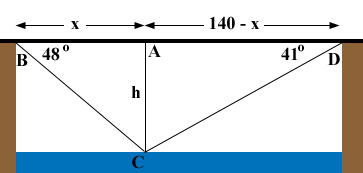SEARCH HOMEMath Central Quandaries & QueriesQuestion from nida, a student: the capilano suspension bridge in north vancouver is the world's highest footbridge of its kind. the bridge is 140m long . from the ends of the bridge the angles of depression of a point on the river under the bridge are 41 degree and 48 dr gee. how high is the bridge above the river to the nearest metreNida,

I drew a diagram of what you described, where h is the height of the bridge above the water. The lengths are in metres.Use a trig function and the triangle ABC to write an equation relating x and h. Repeat but with the triangle DAC. Solve the two equations for h.

PennyMath Central is supported by the University of Regina and The Pacific Institute for the Mathematical Sciences.